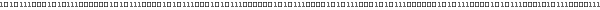# Lesson 1 : Viewing Volume & Viewport• ## Introduction

In this lesson, we will see what is a Viewing Volume and a Viewport. I think this is necessary to understand the Viewing Volume to know how OpenGL works and draw a vertex from the *abstract* 3D space to the window of the screen.

Some informations was found in the OpenGL Programming Guide also known as The Red Book (html version here). This book is really usefull, I encourage you to read it !

• ## Viewing Volume

The 3D space is defined by 3 axis (vectors) : X, Y and Z. These 3 axis creates an axis system (see Tutorial 4 for a representation of this axis system). All points of the 3D space can be expressed as a linear combination of these vectors. A point is point represented by its coordinates (x, y, z), which means x * X + y * Y + z * Z (upper case is a vector, low case is a scalar). The volume of the entire 3D space is infinity.

The Viewing Volume delimites the 3D space by creating a closed volume. Points outside this volume will not appears on the screen.
This particularity explain the first call glTranslate (just after glLoadIdentity) : we go inside the Viewing Volume. At start, we are on the front plane of the viewing volume.

The Viewing Volume can be created with two different kind of projections :
Perspective Projection  Render object affected by the distance, a car is seen smaller when it move away.
Orthographic Projection Render object NOT affected by the distance like a menu, a text on the screen, 2D objects ...

• ### Perspective Projection

With this kind of projection, the viewing volume shape is a truncated pyramid (its top is cutted).

This shape is abtained by 6 cutting planes : front, back, right, left, top and bottom planes (planes at the volume boundary). These planes are commonly called Clipping Planes. These planes can be created with the aid of the method :
glu.gluPerspective(fovy, aspect, near, far)
The fovy parameter is the angle between the plane that goes downwards and the plane that goes upwards.
The aspect ratio is generaly the aspect ratio of the window (window is the region of the screen in which the scene is rendered).

Each parameters are represented in this picture :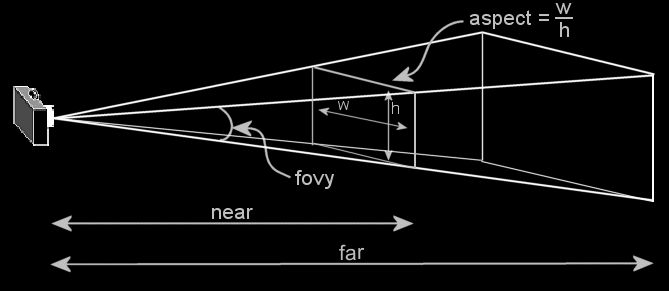Picture Copyrith @ Addison-Wesley Publishing Company

The Viewing Volume looks like :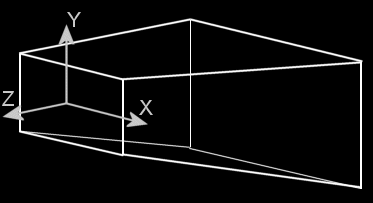Viewing Volume obtained with gluPerspective

The X and Y axis are parallel to the near plane. The origin of the axis system is the center of the near plane. The Z axis is perpendicular to the near plane and points towards the view.
The call glLoadIdentity reset the axis system, like represented in the above picture.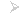Parameter values

Here is an exemple of a viewing volume with perspective projection :
gluPerspective(45.f, width/height, 1.f, 500.f)
The aspect parameter is the ratio between the width and the height of the window (component holding the OpenGL scene).

• ### Orthographic Projection

The Viewing Volume shape in this case is a parallelepiped. The 6 clipping planes delimiting the space can be created with the aid of :
gl.glOrtho(left, right, bottom, top, near, far)

Each parameters are represented in this picture :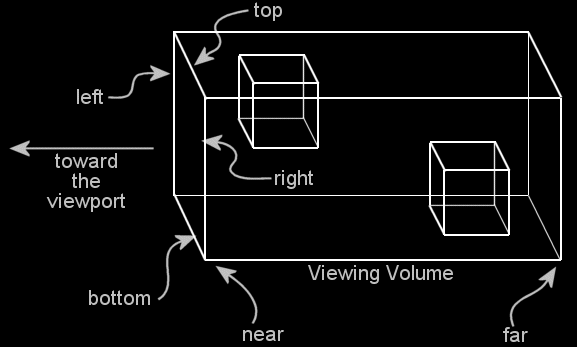Picture Copyrith @ Addison-Wesley Publishing Company

The viewing volume looks like :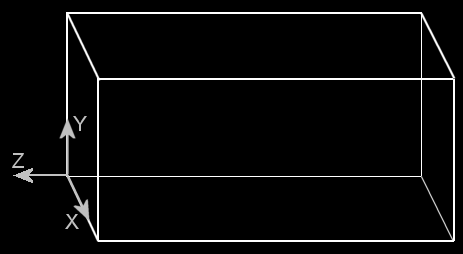Viewing Volume obtained with glOrtho

The axis system is represented in the above picture. In the difference of the perspective projection, the origin is at the lower left corner of the near plane, this means at the bottom left of the window.Values used

Here is an exemple of an orthographic projection :
gl.glOrtho(0, width, 0, height, -1, 1)
The width and height are the dimension of the window.

• ## Clipping Plane

Clipping planes can be used in addition of those used created for the viewing volume. OpenGL define 6 clipping plane GL_CLIP_PLANEi (i goes from 0 to 5).
A clipping plane is a plane that separate the space in two regions. One region is included from the viewing volume, the other is excluded.

In mathematics, a plane is defined by this equation :
a*x+b*y+c*z+d = 0
(x, y, z) are the coordinates of the points in the 3D space. All points that verify this equation are on the plane.
The inequality (< or > instead of =) define the region in each side of the plane.

In OpenGL, a clipping plane is the region defined by this equation :
a*x+b*y+c*z+d >= 0
A clipping plane is creates with the 4 values a, b, c & d likethis :
double[] eq = {a, b, c, d}
gl.glClipPlane(GL_CLIP_PLANEi, eq)    //i can be 0, 1 ...

All points draw , the other are excluded (will not be rendered on the screen).

Like for lights, clipping planes have to be enable to be active :
gl.glEnable(GL_CLIP_PLANEi)           //i can be 0, 1 ...

WARNING: The equation of the clipping plane is related to the axis system at the time when glClipPlane was called (ie the clipping plane region is affected by all previous glTranslate, glRotate and glScale calls). More generally, the clipping plane is affected by the modelview matrix.

• ### How to find the plane equation

To find the plane equation, draw the axis system, the shapes to be clipped and the clipping plane. Then, you pick-up four points on the plane and solve the system of equations :
a*x1+b*y1+c*z1+d = 0
a*x2+b*y2+c*z2+d = 0
a*x3+b*y3+c*z3+d = 0
a*x4+b*y4+c*z4+d = 0
After a small headeach and few hour passed, you will find a, b, c and d (a pocket calculator can be usefull).

• #### Simplification of the system

The previous system of equations can be simplified to this system with 3 equations / 3 unknwon variable :
a*x1+b*y1+c*z1+1 = 0
a*x2+b*y2+c*z2+1 = 0
a*x3+b*y3+c*z3+1 = 0
The system is now a little easier to resolve.

Effectively, the plane equition can be maniplated like this :
a*x + b*y + c*z + d = 0
is equivalent to (division by d) :
a/d*x + b/d*y + c/d*z + d/d = 0/d

The plane equation can be re-formulated as :
a'*x + b'*y + c'*z + 1=0

The values to send to OpenGl are now :
double[] eq = {a', b', c', 1}

• #### Alternative (easier way) : finding the equation by hand !

An alternative is to used the method explained in Tutorial 8.

• ## Viewport

Previously, we have seen how the viewing volume delimitate to 3D space. The Viewport is the region of the screen where this volume is projected.

The viewport can be defined with :
gl.glViewport(x, y, width, height)

Tutorial 7 will show you how to use multiple viewport.

• ## Remarks

When the OpenGL window is reshape, those two properties are updated to respect the new aspect ration and dimension of window.

For an undistored view, the aspect ratio of the viewing volume should be equals to the aspect ratio of the viewport.Back Next Lesson